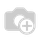• Pricing

# How to sum all values of field based on selected month? (Odoo 13)

Hello,

Suppose I have two Float fields and a date field:

num = fields.Float('Number')
sum_num = fields.Float('Sum all values of the num field')

time_num = fields.Date('Time')

I created the first record and put value: 1.0 in the num field;
num = 1.0
and selected time_num field is :01/01/21 for Ex

and want the result in the sum_num field is 1.0
sum_num = 1.0

Then I created the second record and put value: 2.0 in the num field ;
num = 2.0

and selected time_num field is :01/02/21

and want the result in the sum_num field is 3.0 ;
sum_num = 3.0

And when I create the third record and put value: 3.0 in the num field:
num = 3.0

But I selected for time_num field is : 02/01/21

Then I want the result is : 3.0
sum_num : 3

and so on...

I'd tried to used compute field like this:

sum_num = fields.Float(compute='_sum_num', string='Sum all values of all records of sum field')

@api.depends('num')
def _sum_num(self):
rec_sum = sum(self.search([]).mapped('num')
for rec in self:
rec.sum_num = rec_sum

The compute method working fine but I don't know how to execute it based on the selected month!

Thank you!Hey khanhqn,

May be you can use below compute function instead of your function, it will help you to found record match with based on the selected month.

import calendar

@api.depends('num')
def _sum_num(self):
for rec in self:
last_day = calendar.monthrange(rec.time_num.year, rec.time_num.month)
rec_start = rec.time_num.replace(day=1)
rec_end = rec.time_num.replace(day=last_day)
rec_sum = sum(self.filtered(
lambda x: rec_start < x.time_num and rec_end > x.time_num).mapped('num'))
rec.sum_num = rec_sum

Thanks & Regards,

Email: odoo@aktivsoftware.com

Skype: kalpeshmaheshwari

1 Comment